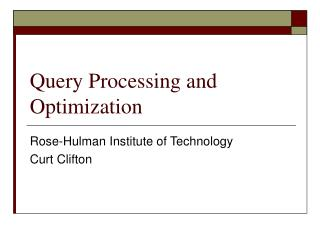DownloadDownload PresentationQuery Processing and Optimization

# Query Processing and Optimization

Télécharger la présentation## Query Processing and Optimization

- - - - - - - - - - - - - - - - - - - - - - - - - - - E N D - - - - - - - - - - - - - - - - - - - - - - - - - - -
##### Presentation Transcript

1. Query Processing and Optimization Rose-Hulman Institute of Technology Curt Clifton

2. Outline • Basic Optimization Approach • Algorithms for Processing Queries • Pipelining • Techniques for Automatic Query Optimization

3. Introduction to Query Processing • What is query optimization? • Typically intermediate form is a query tree

4. From SQL to Relational Algebra • Query block: the basic unit that can be translated into the algebraic operators and optimized • Nested queries become separate query blocks • Aggregate operators in SQL require extended algebra • Example…

5. SELECT LNAME, FNAME FROM EMPLOYEE WHERE SALARY > ( SELECT MAX (SALARY) FROM EMPLOYEE WHERE DNO = 5); SELECT LNAME, FNAME FROM EMPLOYEE WHERE SALARY > C SELECT MAX (SALARY) FROM EMPLOYEE WHERE DNO = 5 πLNAME, FNAME(σSALARY>C(EMPLOYEE)) ℱMAX SALARY(σDNO=5 (EMPLOYEE)) Example Translation

6. What Next? • Queries reduced to query trees in relational algebra • DBMS considers various algorithms for processing query • Rewrites tree to use “best” algorithms • Variety of algorithms exist to solve various query problems

7. Problem: Sorting Huge Datasets • Use external sorting • Phase 1: • Load n pages into memory, as many as fit (a “run”) • Sort them and save back to disk • Repeat until all runs are sorted • Phase 2: • Perform an (n-1)-way merge • One page for “top” of each of n-1 runs • One page for “bottom” of merge results • Repeat until done

8. Problem: Selecting Subset of Rows • Linear search: • Last resort, unless file is small • Binary search: • For ordered data without an index • Using an index for equality comparisons: • Just look up the record

9. Problem: Selecting Subset of Rows • Using a primary index for order comparisons: • Find edge of range using index • Scan from there • Using a secondary index for order comparisons: • Find edge of range using index • Scan leaf nodes of index from there, loading data based on pointers

10. Select With Complex Condition • Simple conjunctive selection: • Pick one condition for which some previous method would work • Use brute force to filter those results based on other conditions • Conjunctive selection with a composite index: • Works if index covers all attributes in the complex condition

11. Select With Complex Condition • Conjunctive selection by intersection of record pointers: • Suppose: • Secondary indexes are several fields in condition • Indexes include record pointers • Then: • Use indexes to get sets of the record pointers for conjuncts • Take intersection of pointer sets • Then retrieve actual records

12. Problem: Joining Two Tables • Nested-loop join (brute force): • Last resort unless tables are small • Single-loop join when one table has index • Loop over one table • Use index to find matches in other table

13. Problem: Joining Two Tables • Sort-merge join when both tables sorted by join attributes • Scan both files matching the records that have the same values for join attributes

14. Problem: Combining Multiple Ops. • Generating and saving temporary files is time expensive • So, avoid constructing temporary results • Pipeline the data through multiple operations: • Pass the result of a previous operator to the next • Page-by-page instead of operation-by-operation • Example…

15. Pipelining Example • SELECT (FName + ' ' + LName) AS NameFROM Employee e JOIN Department d ON e.DNo = d.DNumberWHERE e.Salary < 50000 AND d.Location <> 'Houston' • What are the individual operations for this? • How many ways could this be pipelined?

16. Picking Algorithms and Plans • Heuristics • Cost estimation

17. Using Heuristics • Uses pattern matching to transform parts of query tree to a “best” shape • Patterns based on transformations that are likely to be more efficient: • E.g., Apply selection before applying join • Why is that likely (naively) to be more efficient?

18. Cost-based Optimization • Estimate the costs of a variety of different versions of the query based on:

19. Cost-based Optimization • Estimate the costs of a variety of different versions of the query based on: • Available indexes • Specificity of conditions • Statistics on data • Disk speed • Memory available • Block and record sizes • Index blocking factors

20. Issues in Cost-based Optimization • Accuracy of statistics • Cost of calculating costs • Accuracy of estimates of disk speed, memory available • Shear number of possible execution strategies

21. Which is Used? • Cost-based optimization is “taking over” • SQL Server uses cost-based optimization • Does NOT try to minimize total cost!

22. Which is Used? • Cost-based optimization is “taking over” • SQL Server uses cost-based optimization • Does NOT try to minimize total cost! • Tries to minimize time to initial results